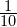# Splitting data into a Training set and a Validation set

Hi guys,

In a machine learning project, after crawling or collecting data, we have to split it into at least 2 parts: training and validation data. The training set is used to build predictive models, while the validation set is for validating the performance of those models.

Rules for splitting

While splitting data, we should adhere to the following 2 rules:

• Both training and validation sets have to well-represent the whole dataset. If the training data does not cover all the traits of the whole data, your models will probably underfit, while if the same happens with validation data, your models’ performance will be assessed incorrectly. The validation set should not have different characteristics from the training set, except for the cases when we do it on purpose to observe how the models handle uncertainty.
• The validation set has to be large enough so that it has statistical power.

Good practices

Normally, the ratio of data points for training and validation is often 7:3 or 8:2.

K-fold cross-validation is a good practice, in which cases the ratio is often 9:1 or even leave-one-out.

A 9:1 ratio corresponds to 10-fold cross-validation, meaning we break the whole dataset into 10 equal parts (folds). Each time we train the model on 9 parts and validate on the remaining part, 10 times.

A leave-one-out strategy is n-fold cross-validation, with n is the size of the whole dataset. Each time we take only 1 sample for validation. Leave-one-out is powerful but only practical if the data size is small or the model is very simple (thus fast to train and validate).

After choosing the number of folds for your cross-validation strategy, let’s move on to determining how the data samples are distributed to each part (fold). There are 2 essential types of methods for this: Simple cross-validation and Stratified cross-validation.

• Simple cross-validation: we just randomly pick samples to put into each part, ensuring that the sizes of all the parts are equal.
• Stratified cross-validation: the process is also random, but there is one more constraint that: we select a column and require that each part must have the same number of samples for each of the values of that column. This is most useful when our dataset is imbalanced, says our dependent variable is binary and 99% of data is positive and only 1% is negative. If we use simple cross-validation with 10-fold, it may happen that 1 part has only positive data and no negative data, which causes damages when we use it as the validation set and the other as the training set. Instead, we can use stratified cross-validation to ensure each of the 10 parts containsnegative samples.

Another important point is: usually when your data is not too small, you should consider making testing set alongside training and validation set. A separate testing set would help you gauss your models’ performance more objectively and accurately. A common ratio for training:validation:testing is 60:20:20.

In some cases, the ratios I introduced above may be changed. For example, if your dataset is very large, you have 10.000.000 diversified samples, the ratio could be 99:0.5:0.5 since 0.5% of 10.000.000 is 50.000, which is large enough for making statistical inferences.

Sklearn’s splitting functions

Sklearn has many pre-built functions to support users on this task. The full list can be found here. Below, I list out the most common ones.

References: